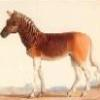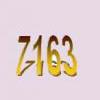# Search by Topic

#### Resources tagged with Prime factors similar to Can it Be:

Filter by: Content type:
Age range:
Challenge level:

### There are 12 results

Broad Topics > Numbers and the Number System > Prime factors### Can it Be

##### Age 16 to 18 Challenge Level:### A Biggy

##### Age 14 to 16 Challenge Level:

Find the smallest positive integer N such that N/2 is a perfect cube, N/3 is a perfect fifth power and N/5 is a perfect seventh power.### Why 24?

##### Age 14 to 16 Challenge Level:

Take any prime number greater than 3 , square it and subtract one. Working on the building blocks will help you to explain what is special about your results.### Factoring a Million

##### Age 14 to 16 Challenge Level:

In how many ways can the number 1 000 000 be expressed as the product of three positive integers?### Em'power'ed

##### Age 16 to 18 Challenge Level:

Find the smallest numbers a, b, and c such that: a^2 = 2b^3 = 3c^5 What can you say about other solutions to this problem?### Rarity

##### Age 16 to 18 Challenge Level:

Show that it is rare for a ratio of ratios to be rational.### Factorial Fun

##### Age 16 to 18 Challenge Level:

How many divisors does factorial n (n!) have?### Different by One

##### Age 14 to 16 Challenge Level:

Make a line of green and a line of yellow rods so that the lines differ in length by one (a white rod)### Fac-finding

##### Age 14 to 16 Challenge Level:

Lyndon chose this as one of his favourite problems. It is accessible but needs some careful analysis of what is included and what is not. A systematic approach is really helpful.### Public Key Cryptography

##### Age 16 to 18

An introduction to the ideas of public key cryptography using small numbers to explain the process. In practice the numbers used are too large to factorise in a reasonable time.### Some Cubes

##### Age 16 to 18 Challenge Level:

The sum of the cubes of two numbers is 7163. What are these numbers?### There's Always One Isn't There

##### Age 14 to 16 Challenge Level:

Take any pair of numbers, say 9 and 14. Take the larger number, fourteen, and count up in 14s. Then divide each of those values by the 9, and look at the remainders.